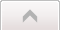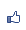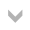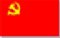共產黨論壇 市長：安津 　副市長： 加入本城市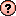｜推薦本城市｜加入我的最愛｜訂閱最新文章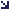本城市首頁討論區精華區投票區影像館推薦連結公告區訪客簿市政中心 (0)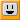開方運算 瀏覽499｜回應0｜推薦0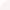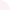亨利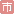等級：8 留言｜加入好友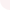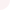# 直式開方法開平方根

《九章算術》〈少廣〉卷中有問：

(a+b)2=a2+2ab+b2=a2+b(2a+b)(a+b)2=a2+2ab+b2=a2+b(2a+b)

(a+b+c)2=a2+b2+c2+2ab+2ac+2bc=a2+b(2a+b)+c(2a+b+c)(a+b+c)2=a2+b2+c2+2ab+2ac+2bc=a2+b(2a+b)+c(2a+b+c)

1. 將 5522555225 從個位數開始二位一撇作記號（定位），由此知所求平方根為三位數 a+b+ca+b+c2. 猜測最接近 55 的平方數為 2(a=200)2(a=200)，從 5522555225 中減去 2002=400002002=40000，剩下 15225152253. 將 200200 自己加一次（22倍），考慮下一位數 bb，要接近而不超過 1522515225，得 b=30b=30
從 1522515225 中減去 30×430=1290030×430=12900，剩下 232523254. 將 3030 再加一次（22倍），併入之前的結果得 460460，考慮下一位數 cc
要接近 23252325 而不超過，得 c=5c=5，從 23252325 中減去 5×465=23255×465=2325，餘數為 00得到 52580235.1152580≈235.11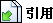引用網址：http://city.udn.com/forum/trackback.jsp?no=50434&aid=5729060